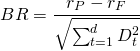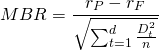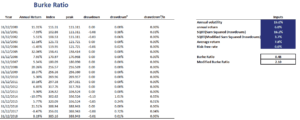# Burke ratio (BR)

The Burke ratio (BR) is a drawdown-based measure of risk-adjusted performance. The measure was first proposed by Burke in 1994 in a paper with the title Burke (1994) “A sharper Sharpe ratio”. To calculate the BR, we should first be able to calculate the drawdown of an investment. We discuss how to calculate the drawdowns on the page drawdown.

Once we are familiar with the concept of drawdowns, the Burke metric is fairly straightforward to calculate. On this page, we discuss the Burke, justify the definition and formula, and discuss an example where we first calculate drawdowns. Finally, we include an Excel spreadsheet that allows you to calculate the Burke ratio for a given return series.

## Burke ratio definition

The BR is similar to the Sharpe ratio in that the BR is also a measure of risk-adjusted performance. Thus, as with the Sharpe ratio, the Burke ratio adjusts performance in the numerator by dividing it by risk. However, instead of using standard deviation in the denominator, we use drawdowns as a measure of risk.

## Burke ratio formula

The Burke ratio formula is the followingwhere d is number of drawdowns, rP is the portfolio return, rF is the risk free rate and Dt the t-th drawdown. Clearly, the ratio looks a bit like the Sharpe ratio, but with a different denominator.

## Modified Burke ratio

In addition to the standard ratio, some investors also use a modified version of the ratio. The modified Burke is defined as followswhere n is the number of data points.  Thus, the modified Burke ratio adjusts the original ratio by taking into consideration the number of drawdowns that has occured over the period we are analyzing.

## Example

Next, we discuss a Burke ratio example to see how we can easily implement the above formula using a spreadsheet. The following figure illustrates the main steps needed to calculate the BR and MBR. From the figure, it is clear that to calculate the measure, we only need a time-series of the fund’s returns.The Excel spreadsheet pictured above can be downloaded at the bottom of the page.

## Summary

We discussed the Burke ratio, a measure of risk-adjusted performance that is an alternative to the Sharpe ratio. The ratio is often used to assess the performance of hedge funds, who promise to generate positive absolute returns. For these funds, controlling downside risk is very important. Other measures that use downside risk are the Omega ratio, Sterling ratio, Kappa ratio, and the Calmar ratio. Often, it is best to calculate a few of these measures simultaneously, because they tend to look at different aspects of downside risk.

### Burke metric calculator

Want to have an implementation in Excel? Download the Excel file: Burke Ratio template